Olympiad Test: The Triangle and Its Properties

# Olympiad Test: The Triangle and Its Properties

Test Description

## 20 Questions MCQ Test Mathematics (Maths) Class 7 | Olympiad Test: The Triangle and Its Properties

Olympiad Test: The Triangle and Its Properties for Class 7 2023 is part of Mathematics (Maths) Class 7 preparation. The Olympiad Test: The Triangle and Its Properties questions and answers have been prepared according to the Class 7 exam syllabus.The Olympiad Test: The Triangle and Its Properties MCQs are made for Class 7 2023 Exam. Find important definitions, questions, notes, meanings, examples, exercises, MCQs and online tests for Olympiad Test: The Triangle and Its Properties below.
Solutions of Olympiad Test: The Triangle and Its Properties questions in English are available as part of our Mathematics (Maths) Class 7 for Class 7 & Olympiad Test: The Triangle and Its Properties solutions in Hindi for Mathematics (Maths) Class 7 course. Download more important topics, notes, lectures and mock test series for Class 7 Exam by signing up for free. Attempt Olympiad Test: The Triangle and Its Properties | 20 questions in 20 minutes | Mock test for Class 7 preparation | Free important questions MCQ to study Mathematics (Maths) Class 7 for Class 7 Exam | Download free PDF with solutions
 1 Crore+ students have signed up on EduRev. Have you?
Olympiad Test: The Triangle and Its Properties - Question 1

### A triangle in which two sides are of equal lengths is called _______________.

Olympiad Test: The Triangle and Its Properties - Question 2

### The sum of the lengths of any two sides of a triangle is _____________ the third side of the triangle.

Olympiad Test: The Triangle and Its Properties - Question 3

### In the Pythagoras property, the triangle must be ___________ .

Olympiad Test: The Triangle and Its Properties - Question 4

Find the value of x in this figure.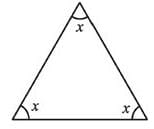Olympiad Test: The Triangle and Its Properties - Question 5

Find the value of x in given figure.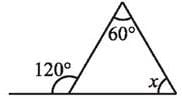Detailed Solution for Olympiad Test: The Triangle and Its Properties - Question 5

Exterior angle is equal to sum of interior opposite angles so,
120 = 60+x
x = 60°

Olympiad Test: The Triangle and Its Properties - Question 6

In ΔPQR, PD is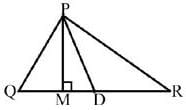Detailed Solution for Olympiad Test: The Triangle and Its Properties - Question 6

Median is a line joining the vertex and middle of the opposite side, and hence dividing the triangle in two equal parts. So PD is the median.

Olympiad Test: The Triangle and Its Properties - Question 7

The value of x in the adjoining figure is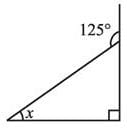Olympiad Test: The Triangle and Its Properties - Question 8

What is the value of x in the below figure.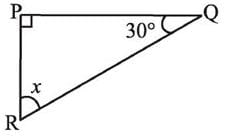Olympiad Test: The Triangle and Its Properties - Question 9

Find the value of unknown x in the adjoining figure.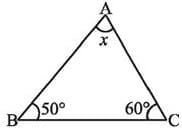Olympiad Test: The Triangle and Its Properties - Question 10

What is the measure of angle x ?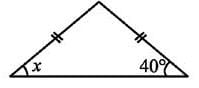Olympiad Test: The Triangle and Its Properties - Question 11

ΔABC is right-angled at C. If AC = 5 cm and BC = 12 cm find the length of AB.

Detailed Solution for Olympiad Test: The Triangle and Its Properties - Question 11

AB2=AC2+BC2=52+122=25+144 = 169
AB=13 cm

Olympiad Test: The Triangle and Its Properties - Question 12

ΔPQR is a triangle right-angled at P. If PQ = 3 cm and PR = 4 cm, find QR.

Detailed Solution for Olympiad Test: The Triangle and Its Properties - Question 12

Using pythagoras theorem,
QR= PR+ PQ2
42+32
=16+9=25
QR = 5cm

Olympiad Test: The Triangle and Its Properties - Question 13

Which is the longest side in the triangle PQR right angled at P?

Olympiad Test: The Triangle and Its Properties - Question 14

Which is the longest side in the triangle ABC right angled at B?

Olympiad Test: The Triangle and Its Properties - Question 15

Which is the longest side of a right triangle?

Olympiad Test: The Triangle and Its Properties - Question 16

What is the measure of angles x?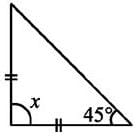Detailed Solution for Olympiad Test: The Triangle and Its Properties - Question 16

Since the two sides are equal, the angles opposite to them are also equal. So x+45° + 45° = 180°, x = 90°

Olympiad Test: The Triangle and Its Properties - Question 17

The value of x in the adjoining figure is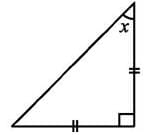Olympiad Test: The Triangle and Its Properties - Question 18

A triangle in which all three sides are of equal lengths is called _________.

Olympiad Test: The Triangle and Its Properties - Question 19

Find angle x in below figure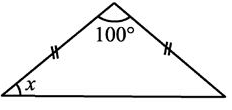Olympiad Test: The Triangle and Its Properties - Question 20

Find angle x in below figure.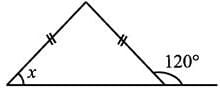Detailed Solution for Olympiad Test: The Triangle and Its Properties - Question 20

Since the two sides are equal, the angles opposite to them are also equal to the adjacent angle of 120° which is equal to 180° - 120° =60° is equal to x. So x = 60°

## Mathematics (Maths) Class 7

168 videos|276 docs|45 tests
 Use Code STAYHOME200 and get INR 200 additional OFF Use Coupon Code